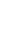# What is Fixed Interest Rate? - Definition, Calculation, Advantages

Updated:2 Sep, 2022

Fixed Interest Rates are those interest rates that do not change with time, a person can repay his liability to the insurer without paying much interest. This type of interest helps take loans but not for investment purposes. Also, this is helpful for borrower who wants certainty in their loan interest.

• What are Fixed Interest Rates
• Calculation of Fixed Interest Rates
• Comparison between Fixed Interest Rates and Variable (Floating) Interest Rates
• Example

## What are Fixed Interest Rates?

When you borrowed money an interest rate is charged on the borrowed money and generally expressed as an annual percentage. Fixed Interest Rates are popular among those who want certainty in repaying the borrowed sum. For example, if you borrow a sum of 1 lakh for 10 years at 5 percent interest then this interest will be constant throughout the 10 years of your loan tenure. These fixed interest rates are generally higher than the variable interest rate as they cover less risk on the factuality of the loan interest.

## Calculation of Fixed Interest Rates

Calculation of Fixed interest rate is very simple, only a few things are required to be known that is: Principal amount (the borrowed amount), Interest rate, and period of the borrowed money. The formula goes simple- Principal x Rate of interest x time.

``````Suppose the tenure of the loan is 5 years and the loan amount is 15000 at a fixed rate of 5%. Find the interest to be given in 5 years.

Sol: Here The Principal = 15000, Rate of Interest is 5%, and the Time =5 years.
Therefore, Interest = 15000x5%x5= 3750.``````
``````Consider another scenario, where you buy a car loan of Rs 500000 for 5 years at a rate of 2%. Let's find the Amount to be paid back to the lender.
Sol: Here, we have to calculate the loan amount as well as the total amount to be paid back.

Given, Principal= Rs 500000, Rate= 2% and Time = 5 Years.
Fixed Interest = 500000x2%x5= 10000 Also the amount to be paid back will be 500000+10000= Rs 510000.``````

### Advantages (Merits) of Fixed Interest:

• Low Rates: When the interest rates are low then it is the best option to go for Fixed interest loans
• Predictability: Since the interest is the same throughout, monthly expenses can be managed.
• Cost of Borrowing: Since the interest rate is fixed it is easier to calculate the cost of the loan.
• Increasing Rates: If the interest rates increase overnight the borrower will not be affected.

### Disadvantages (Demerits) of Fixed Interest:

• Higher than Floating Rates: Depending on the overall interest it can be found that it is higher than the floating interest rates.
• Refinancing: Shifting from fixed to floating interest can be time-consuming and also, and closing costs may be high
• Higher Repayments: If the interest rate falls but you still have to pay more interest than this.

## Fixed Interest Vs Floating Interest:

Floating Interests Rates: As the name suggests, Floating Interests Rates are the ones that change with the period of time, because they are affected by the conditions of economic and financial markets.

When looking at loans you notice that some are listed with a fixed interest rate and others with a variable interest rate what's the difference is one better than the other which one is right for you, answer all of those questions and more whether you're looking at purchasing a home and taking out a mortgage or whether comparing loan options or maybe just for evaluating current debt that all is something important to be kept in our mind whether you've got a fixed or a variable interest rate given the option which of these should you choose

Consider a scenario, For Example, Getting the house of your dreams for most people means getting a home loan. If you want the security of knowing exactly what your home loan repayments will be then a fixed interest rate might be for you. With a fixed interest rate you can choose an interest for a fixed period.

This can be a selected term, ranging from six months to seven years. All the payments done by you during this period will be the same. You could break your fixed term but you might be charged. Your fixed rate can't be affected by interest rate changes. So whether interest rates go up or go down, your repayments stay the same until the end of the fixed period when you could change to a new fixed rate or move to a floating rate of your choice, or choose a combination of the two. If you do nothing, the bank automatically rolls you to its standard floating rate.

Lastly, If you find that interest rates are cheaper at that time, go for Fixed otherwise float, It also depends on the risk-taking capacity of the person, the Floating rate comes with more risk. Also, always take a loan from a reputed and trusted source and read the loan papers and understand the terms that are not clear to you. Furthermore, it is okay to know how interest works, but do not take an unnecessary loan just for trial.